## Protons can be accelerated to speeds near that of light in particle accelerators. Estimate the wavelength (in nm) of such a proton moving at

Question

Protons can be accelerated to speeds near that of light in particle accelerators. Estimate the wavelength (in nm) of such a proton moving at 2.91 × 108 m/s (mass of a proton = 1.673 × 10−27 kg). Enter your answer in scientific notation.

in progress 0
5 months 2021-08-17T06:54:50+00:00 1 Answers 12 views 0

The wavelength is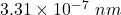.

Explanation:

Given:

Rest mass of the proton (m₀) = 1.673 × 10⁻²⁷ kg

Velocity of the proton (v) = 2.91 × 10⁸ m/s

Wavelength of the proton (λ) = ?

Here, the proton is accelerated nearly to speed of light. So, the mass is no more a constant value. It’s value is calculated using the relativistic mass equation which is given as: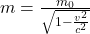Plug in the values given and solve for ‘m’. This gives,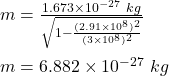In order to determine the wavelength of proton, we have to make use of the formula of de Broglie wavelength.

The de Broglie wavelength is given as: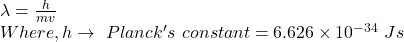Now, plug in the values given and solve for λ. This gives,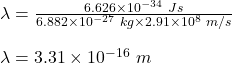Now, 1 m = 10⁹ nm

Therefore,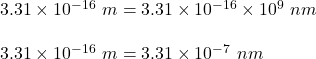Hence, the wavelength is 3.31 × 10⁻⁷ nm.